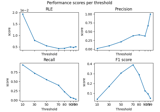# mne.simulation.metrics.f1_score#

mne.simulation.metrics.f1_score(stc_true, stc_est, threshold='90%', per_sample=True)[source]#

Compute the F1 score, also known as balanced F-score or F-measure.

The F1 score can be interpreted as a weighted average of the precision and recall, where an F1 score reaches its best value at 1 and worst score at 0. The relative contribution of precision and recall to the F1 score are equal. The formula for the F1 score is:

```F1 = 2 * (precision * recall) / (precision + recall)
```

Threshold is used first for data binarization.

Parameters:
stc_trueinstance of (Vol|Mixed)SourceEstimate

The source estimates containing correct values.

stc_estinstance of (Vol|Mixed)SourceEstimate

The source estimates containing estimated values e.g. obtained with a source imaging method.

threshold

The threshold to apply to source estimates before computing the f1 score. If a string the threshold is a percentage and it should end with the percent character.

per_sample`bool`

If True the metric is computed for each sample separately. If False, the metric is spatio-temporal.

Returns:
metric`float` | `array`, shape (n_times,)

The metric. float if per_sample is False, else array with the values computed for each time point.

Notes

New in version 1.2.

## Examples using `mne.simulation.metrics.f1_score`#Compare simulated and estimated source activity

Compare simulated and estimated source activity